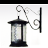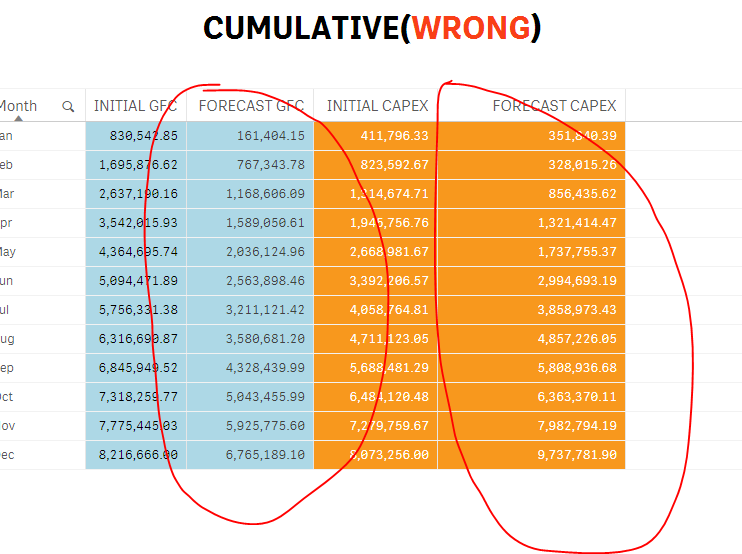Announcements
You can succeed best and quickest by helping others to succeed. Join the conversation.
cancel
Showing results for
Did you mean:Creator III

## ?

.

1 Solution

Accepted SolutionsMVP

Try this

RangeSum(Above(If(Month(Months) <= Month(Today()), (Sum({\$<[type]={'Actual GFC'}>} value)), (sum({\$<[type]={'Forecast GFC'}>} value))), 0, RowNo()))

RangeSum(Above(If(Month(Months) <= Month(Today()), (Sum({\$<[type]={'Actual CAPEX'}>} value)), (Sum({\$<[type]={'Forecast CAPEX'}>} value))), 0, RowNo()))

So, instead of rangesumming the individual expression, rangesum the whole if statement8 RepliesCreator III
Author

/.MVP

Looks good... is it not working for you?Creator III
Author

xMVP

You are saying we are in March? Are we really in March? I am not sure I understand how can we be in March when it is only January? I am not sure I follow what is incorrect here.Creator III
Author

aMVP

Can you just provide me the numerical output you are looking to get in a Excel file? I understand the logic, but I am not sure what isn't working.Creator III
Author

zMVP

Try this

RangeSum(Above(If(Month(Months) <= Month(Today()), (Sum({\$<[type]={'Actual GFC'}>} value)), (sum({\$<[type]={'Forecast GFC'}>} value))), 0, RowNo()))

RangeSum(Above(If(Month(Months) <= Month(Today()), (Sum({\$<[type]={'Actual CAPEX'}>} value)), (Sum({\$<[type]={'Forecast CAPEX'}>} value))), 0, RowNo()))

So, instead of rangesumming the individual expression, rangesum the whole if statementCommunity Browser